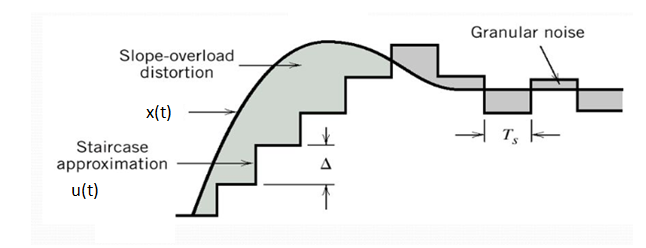# What is Slope Overload distortion and Granular Noise in Delta Modulation and how it is removed in ADM

The delta modulation has two major drawbacks as under :

2. Granular or idle noise

Now, we will discuss these two drawbacks in detail.

This distortion arises because of large dynamic range of the input signal.Fig.1: Quantization Errors in Delta Modulation

We can observe from fig.1 , the rate of rise of input signal x(t) is so high that the staircase signal can not approximate it, the step size ‘Δ’ becomes too small for staircase signal u(t) to follow the step segment of x(t).

Hence, there is a large error between the staircase approximated signal and the original input signal x(t).

This error or noise is known as slope overload distortion .

To reduce this error, the step size must be increased when slope of signal x(t) is high.

### 2. Granular or Idle Noise

Granular or Idle noise occurs when the step size is too large compared to small variation in the input signal.

This means that for very small variations in the input signal, the staircase signal is changed by large amount (Δ) because of large step size.

Fig.1 shows that when the input signal is almost flat , the staircase signal u(t) keeps on oscillating by ±Δ around the signal.

The error between the input and approximated signal is called granular noise.

The solution to this problem is to make the step size small .

### Solution

In order to overcome the quantization errors due to slope overload and granular noise, the step size (Δ) is made adaptive to variations in the input signal x(t).

Particularly in the steep segment of the signal x(t), the step size is increased. And the step is decreased when the input is varying slowly.